International Tables for Crystallography (2010). Vol. B, ch. 2.4, pp. 282-296   | 1 | 2 | https://doi.org/10.1107/97809553602060000766

Contents

• 2.4. Isomorphous replacement and anomalous scattering  (pp. 282-296)
• 2.4.1. Introduction  (p. 282) | html | pdf |
• 2.4.2. Isomorphous replacement method  (pp. 282-283) | html | pdf |
• 2.4.2.1. Isomorphous replacement and isomorphous addition  (pp. 282-283) | html | pdf |
• 2.4.2.2. Single isomorphous replacement method  (p. 283) | html | pdf |
• 2.4.2.3. Multiple isomorphous replacement method  (p. 283) | html | pdf |
• 2.4.3. Anomalous-scattering method  (pp. 284-287) | html | pdf |
• 2.4.3.1. Dispersion correction  (p. 284) | html | pdf |
• 2.4.3.2. Violation of Friedel's law  (pp. 284-285) | html | pdf |
• 2.4.3.3. Friedel and Bijvoet pairs  (p. 285) | html | pdf |
• 2.4.3.4. Determination of absolute configuration  (pp. 285-286) | html | pdf |
• 2.4.3.5. Determination of phase angles  (p. 286) | html | pdf |
• 2.4.3.6. Anomalous scattering without phase change  (p. 286) | html | pdf |
• 2.4.3.7. Treatment of anomalous scattering in structure refinement  (p. 287) | html | pdf |
• 2.4.4. Isomorphous replacement and anomalous scattering in protein crystallography  (pp. 287-293) | html | pdf |
• 2.4.4.1. Protein heavy-atom derivatives  (p. 287) | html | pdf |
• 2.4.4.2. Determination of heavy-atom parameters  (pp. 287-288) | html | pdf |
• 2.4.4.3. Refinement of heavy-atom parameters  (pp. 289-290) | html | pdf |
• 2.4.4.4. Treatment of errors in phase evaluation: Blow and Crick formulation  (pp. 290-291) | html | pdf |
• 2.4.4.5. Use of anomalous scattering in phase evaluation  (p. 291) | html | pdf |
• 2.4.4.6. Estimation of r.m.s. error  (pp. 291-292) | html | pdf |
• 2.4.4.7. Suggested modifications to Blow and Crick formulation and the inclusion of phase information from other sources  (pp. 292-293) | html | pdf |
• 2.4.4.8. Fourier representation of anomalous scatterers  (p. 293) | html | pdf |
• 2.4.5. Anomalous scattering of neutrons and synchrotron radiation. The multiwavelength method  (pp. 293-294) | html | pdf |
• 2.4.5.1. Neutron anomalous scattering  (pp. 293-294) | html | pdf |
• 2.4.5.2. Anomalous scattering of synchrotron radiation  (p. 294) | html | pdf |
• References | html | pdf |
• Figures
• Fig. 2.4.2.1. Vector relationship betweenand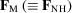(p. 283) | html | pdf |
• Fig. 2.4.2.2. Relationship between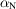,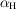and(p. 283) | html | pdf |
• Fig. 2.4.2.3. Harker construction when two heavy-atom derivatives are available  (p. 284) | html | pdf |
• Fig. 2.4.3.1. Variation of ( a )and ( b )as a function of atomic number for Cuand Moradiations  (p. 284) | html | pdf |
• Fig. 2.4.3.2. Vector diagram illustrating the violation of Friedel's law when(p. 285) | html | pdf |
• Fig. 2.4.3.3. A composite view of the vector relationship between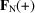and(p. 285) | html | pdf |
• Fig. 2.4.4.1. Distribution of intersections in the Harker construction under non-ideal conditions  (p. 290) | html | pdf |
• Fig. 2.4.4.2. Vector diagram indicating the calculated structure factor,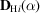, of the i th heavy-atom derivative  (p. 290) | html | pdf |
• Fig. 2.4.4.3. The probability distribution of the protein phase angle  (p. 290) | html | pdf |
• Fig. 2.4.4.4. Harker construction using anomalous-scattering data from a single derivative  (p. 291) | html | pdf |
• Tables
• Table 2.4.3.1. Phase angles of different components of the structure factor in space group P 222  (p. 285) | html | pdf |